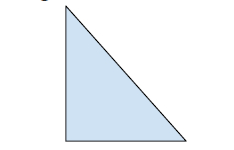# Find the dimensions of Right angled triangle in C++

C++Server Side ProgrammingProgramming

#### C in Depth: The Complete C Programming Guide for Beginners

45 Lectures 4.5 hours

#### Practical C++: Learn C++ Basics Step by Step

Most Popular

50 Lectures 4.5 hours

#### Master C and Embedded C Programming- Learn as you go

66 Lectures 5.5 hours

In this problem, we are given two values H and A, denoting the hypotenuse and area of a right angled triangle. Our task is to find the dimensions of the Right angled triangle.

Right Angled Triangle is a special type of triangle in which two sides intersect at right angles.Fig : Right Angle Triangle

Let's take an example to understand the problem,

Input : H = 7 , A = 8
Output : height = 2.43, base = 6.56

## Solution Approach

Solution to this problem can be found by using the mathematical formula for the values. And lets derive them here,

$A\:=\:1/2^*h^*b$

$H^2\:=\:h^2\:+\:b^2$

Using the formula,

$(h+b)^2\:=\:h^2+b^2+2^*h^*b$

$(h+b)^2\:=\:H^2+4^*A$

$(h+b)\:=\:\sqrt(H^2+4^*A)$

Similarly using the formula,

$(h-b)^2\:=\:h^2+b^2-2^*h^*b$

$(h-b)^2\:=\:H^2-4^*A$

$(h-b)^2\:=\:\sqrt(H^2-4^*A)$

Here, we have two equations,

On adding both we have

$h-b+h-b\:=\:\sqrt(H^2-4^*A)\:+\:\sqrt(H2-4^*A)$

$2h\:=\:(\sqrt(H^2-4^*A))\:+\:(\sqrt(H^2-4^*A))$

$h\:=\:1/2^*(\sqrt(H^2-4^*A))\:+\:(\sqrt(H^2-4^*A))$

On subtracting both we get,

$h-b-h+b\:=\:\sqrt(H^2-4^*A)-\sqrt(H^2-4^*A)$

$2b\:=\:(\sqrt(H^2-4^*A)\:-\:\sqrt(H^2-4^*A))$

$b\:=\:1/2^*(\sqrt(H^2-4^*A)\:-\:\sqrt(H^2-4^*A))$

Applying both formulas to get the values of b and h.

## Example

Program to illustrate the working of our solution

#include <iostream>
#include <math.h>
using namespace std;
void findAllDismensionsRightTriangle(int H, int A) {
if (H * H < 4 * A) {
cout<<"Not Possible\n";
return;
}
float val1 = (float)sqrt(H * H + 4 * A);
float val2 = (float)sqrt(H * H - 4 * A);
float b = (float)(val1 + val2) / 2.0;
float p = (float)(val1 - val2) / 2.0;
cout<<"Perpendicular = "<<p<<endl;
cout<<"Base = "<<b;
}
int main() {
int H = 7;
int A = 8;
cout<<"The dimensions of the triangle are : \n";
cout<<"Hypotenuse = "<<H<<endl;
findAllDismensionsRightTriangle(H, A);
return 0;
}

## Output

The dimensions of the triangle are :
Hypotenuse = 7
Perpendicular = 2.43845
Base = 6.56155